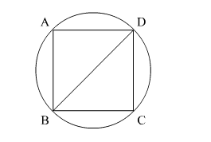# Find the area of the circle in which a squareQuestion:

Find the area of the circle in which a square of area $64 \mathrm{~cm}^{2}$ is inscribed. [Use $\left.\pi=3.14\right]$

Solution:We have given area of the square.

$\therefore$ side $^{2}=64$

$\therefore$ side $=8$

Now we will find the diameter of the square.

$\therefore$ diagonal $=\sqrt{2} \times$ side

$\therefore$ diagonal $=\sqrt{2} \times 8$

$\therefore$ diagonal $=8 \sqrt{2}$

We know that diagonal of the square is same as the diameter of the circle.

$\therefore$ diameter $=8 \sqrt{2}$

$\therefore$ radius $=4 \sqrt{2}$

Now we will find the area of the circle as shown below.

$\therefore$ area of the circle $=\pi \times r^{2}$

$\therefore$ area of the circle $=\pi \times 4 \sqrt{2} \times 4 \sqrt{2}$

$\therefore$ area of the circle $=3.14 \times 16 \times 2$

$\therefore$ area of the circle $=3.14 \times 32$

$\therefore$ area of the circle $=100.48$

Therefore, area of the circle is $100.48 \mathrm{~cm}^{2}$